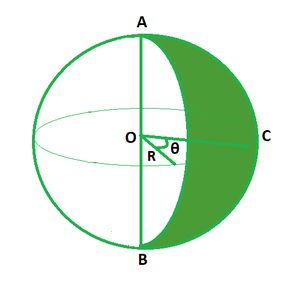Open in App
Not now

# Spherical Wedge and Spherical Lune Formula

• Last Updated : 28 Apr, 2022

A spherical wedge is a solid created by rotating a semicircle about its diameter with fewer than 360o. The region on a sphere bordered by two half circles that intersect at antipodal points is known as a spherical lune. It is defined as the curved surface area of the wedge. The term antipodal refers to that point on the surface of sphere which is diametrically opposed to it.In the above diagram, the region AOB is the spherical wedge and the shaded region is called as a spherical lune. The shape of lune resembles a curve and it has only curved surface area.

### Spherical Wedge and Spherical Lune Formula

A spherical wedge is comparable to a sphere and a spherical lune is similar to a spherical surface. Ratios and proportions are used to obtain formulas for wedge and lune.

Volume of spherical wedge

The ratio of volume of wedge to the central angle θ equals the volume of sphere per revolution. The volume of a wedge is equal to two-third of the product of cube of radius and the central angle (in radians).

Volume of wedge/Angle at center = Volume of sphere/1 revolution

V/θ = 4/3 πR3/360o

V = πR3θdeg/270

Area of lune

The ratio of area of lune to the central angle θ equals the area of sphere per revolution. The area of lune is equal to twice the product of square of radius and the central angle (in radians).

Area of lune/Angle at center = Area of sphere/1 revolution

A/θ = 2πR2/360o

A = πR2θdeg/90

### Sample Problems

Problem 1. Calculate the volume of the spherical wedge if the radius is 5 m and angle of the wedge is π/4 radians.

Solution:

We have, r = 5 and θ = π/4

Using the formula we have,

V = 2/3 R3θ

= 2/3 (53) (π/4)

= 261.66/4

= 65.41 cu. m

Problem 2. Calculate the volume of the spherical wedge if the radius is 7 m and angle of the wedge is π/3 radians.

Solution:

We have, r = 7 and θ = π/3

Using the formula we have,

V = 2/3 R3θ

= 2/3 (73) (π/3)

= 15092/63

= 79.77 cu. m

Problem 3. Calculate the area of the spherical wedge if the radius is 3 m and angle of the wedge is π/4 radians.

Solution:

We have, r = 7 and θ = π/3

Using the formula we have,

A = 2R2θ

= 2 (7) (7) (π/3)

= 2156/21

= 102.66 sq. m

Problem 4. Calculate the area of the spherical wedge if the radius is 10 m and angle of the wedge is π/4 radians.

Solution:

We have, r = 10 and θ = π/4

Using the formula we have,

A = 2R2θ

= 2 (10) (10) (π/4)

= 4400/28

= 157.14 sq. m

Problem 5. Find the central angle of the spherical lune if its radius is 6 m and area is 220 sq. m.

Solution:

We have, A = 220, r = 6.

Using the formula we have,

A = 2R2θ

=> 220 = 2 (6) (6) θ

=>θ = 220/72

Problem 6. Find the central angle of the spherical wedge if its radius is 7 m and volume is 660 sq. m.

Solution:

We have, V = 660, r = 7.

Using the formula we have,

V = 2/3 R3θ

=> 660 = 2/3 (7)3 θ

=> 660 = 228.66θ

=> θ = 660/228.66

Problem 7. Find the area of the spherical wedge if its radius is 12 m and volume is 1320 sq. m.

Solution:

We have, V = 1320, r = 12.

Using the formula we have,

V = 2/3 R3θ

=> 1320 = 2/3 (12)3 θ

=> 3960 = 3456θ

=> θ = 3960/3456